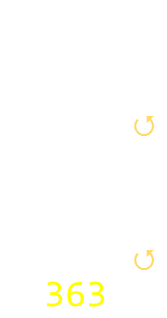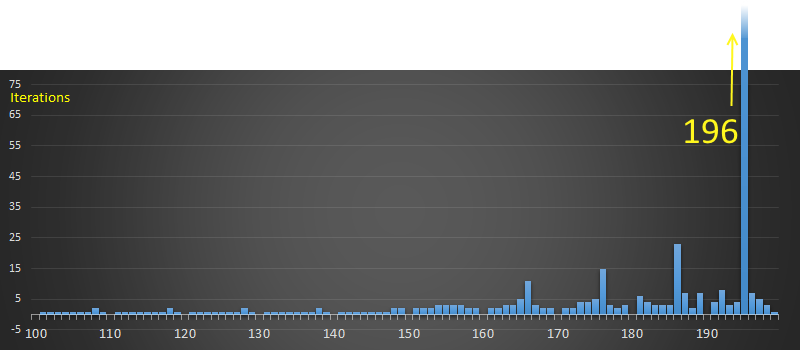﻿ Lychrel Numbers# Lychrel Numbers

 This article is based on a recent Numberphile video. It relates to an interesting property of numbers to eventually turn into palindromes when added to themselves reversed. Here’s how it works: Take any positive integer number. Is the number a palindrome (does it read the same back-to-front)? If yes, stop. If not, write the current number backwards and add this to the current number. This sum is the new number. Goto step 2We keep track of how long it takes for a number to turn palindromic. As you can see from the example above, 39 took two steps. Some numbers (such as 22, 101, 666, 1221 …) are already palindromes, and take zero steps. By definition, all single digit numbers are also palindromes.

Most numbers turn into palindromes in just a couple of steps. e.g. 21 turns into a palindrome in one iteration. Even a larger number, such as 1967, turns into a palindrome with just five steps. Do all numbers turn into palindromes? If so, what numbers take the longest number of steps? What is the most delayed palindrome so far discovered?

 1967 9658 18227 90508 171017 +7691 +8569 +72281 +80509 +710171 9658 18227 90508 171017 881188

### Early Numbers

Below is a chart for the starting seeds of 1-100 (on the x-axis), and a count of the number of iterations for it to become palindromic:All numbers less than 100, (with the exception of 89 and 98), take six or fewer iterations; most take less. It should be no surprise that 89 and 98 take the same number of steps as they are canonical forms of themselves (89 reversed is 98!). They both take 24 steps and the final palindromic number generated is 8813200023188.

89 → 187 → 968 → 1837 → 9218 → 17347 → 91718 → 173437 → 907808 → 1716517 → 8872688 → 17735476 → 85189247 → 159487405 → 664272356 → 1317544822 → 3602001953 → 7193004016 → 13297007933 → 47267087164 → 93445163438 → 176881317877 → 955594506548 → 1801200002107 → 8813200023188

What happens with the next 100 numbers? Here is a graph of the numbers 100-200Something interesting happens. 187 takes 23 steps (one less than 89; but that's not a surprise, because if you look at the sequence above for 89 you see that the second term is 187, and after that they, of course, follow the same chain).

The more interesting feature, however, is 196. It does not quickly form a palindrome. In fact, we don't know if it ever does!

Quite a lot of processing power has been dedicated to researching if 196 eventually becomes a palindrome. How much? Well, using distributed computing, Romain Dolbeau went through a billion iterations to produce a number with 413,930,770 digits, and still no palindrome was seen! (He went on to pass 600 million digits without finding anything).

## Lychrel Numbers

196 is not unique, and other numbers possess this property. Here is list of the first few numbers that do not converge into a palindrome:*

196, 295, 394, 493, 592, 689, 691, 788, 790, 879, 887, 978, 986, 1495, 1497, 1585, 1587, 1675, 1677, 1765, 1767, 1855, 1857, 1945, 1947, 1997, 2494, 2496, 2584, 2586, 2674, 2676, 2764, 2766, 2854, 2856, 2944, 2946, 2996, 3493, 3495, 3583, 3585, 3673, 3675 …

These numbers are given the name of Lychrel Numbers, as an (almost) palindrome of "Cheryl", the girlfriend (now wife!) of Wade VanLandingham, one of the researchers of this phenomenon,

Similar computerized brute force searches to find palindromes on these other Lychrel numbers have been attempted with no success. It's looking likely that these numbers never converge to palindromes, but this property has not yet been mathematically proven.

*Purists, discount many of these numbers as true Lychrel numbers because, for instance, the chains of 196 and 295 both converge to 887 on their second step. If you only want to include pure Lychrel numbers (sometimes called 'seeds'), here is a list of the first few terms:

196, 879, 1997, 7059, 10553, 10563, 10577, 10583, 10585, 10638, 10663, 10668, 10697, 10715, 10728, 10735, 10746, 10748, 10783, 10785, 10787, 10788, 10877, 10883, 10963, 10965, 10969, 10977, 10983, 10985, 12797, 12898, 13097, 13197, 13694 …

(The other potential point of contention between purists is if the palindrome test is performed before the first addition, or after. e.g. If before, obviously 9999 is not Lychrel, but if you require a least one iteration before test, it is, as it has never been found to palindrome after starting).

### Trivia

• Interestingly 89 takes an unusually long time to palindrome. The 24 steps (rendered above), is the largest number of steps (of non-Lyrchel numbers) less than 10,000.

• For numbers less than 10,000 a staggering 29.9% of all numbers turn palindromic in one (or less) iterations.

• For numbers less than 10,000 over 80.5% turn palindromic in four or less iterations.

• Obviously, if a number is Lychrel, so is its palindrome (unless you are a purist, in which case you simply take the smallest as a seed).

• The word 'palindrome' comes from the Greek 'palíndromos', which means "running back again".

• At 10,911 (just after 10,000), the longest delayed palindrome number jumps to requiring 55 steps. This is the most steps needed for any number below 100,000

• Below 1,000,000 the longest delayed palindrome requires 64 steps, and this is 150,296.

### Delayed Palindromes

In addition to searching for (and proving, or disproving Lychrel numbers), researchers have used computers to find the longest delayed palindrome they can (that is not Lychrel). You can find their research here.

An exhaustive test has been performed for smaller digit numbers. The longest found are shown below:

Largest Delay per Digit Set
Digit Set Largest Delay
1-digit numbers
2-digit numbers
3-digit numbers
4-digit numbers
5-digit numbers
6-digit numbers
7-digit numbers
8-digit numbers
9-digit numbers
10-digit numbers
11-digit numbers
12-digit numbers
13-digit numbers
14-digit numbers
15-digit numbers
16-digit numbers
17-digit numbers
18-digit numbers
19-digit numbers
20-digit numbers
2
24
23
21
55
64
96
96
98
109
149
149
188
186
201
197
236
232
261
257

The current largest known delayed palindrome number takes 261 steps to turn the 19-digit number: 1,186,060,307,891,929,990 into the 119 digit palindrome of (A search of all the 20 digit numbers did not reveal a longer delayed number):

445626658789764376224378489766538703888847836625984258559634
36955852489526638748888307835667984873422673467987856626544

### Density

As the length of the starting number changes, so does the chance that a number will be Lychrel. By the time numbers are at 18 diigts of length, over 90% of them turn into Lychrel numbers and never convert to palindromes.The longer a number gets, the smaller the chance that a palindrome will be generated (a palindrome can only be formed if there is no carry when two digits are added). Considering how large the potential Lychrel numbers have been tested to, even if not proved yet, it's incredibly unlikely they will ever converge to palindromes.

### Applications

Sadly, other than interest, and an excuse to write code, I can think of no practical used for Lychrel numbers!

You can find a complete list of all the articles here.Click here to receive email alerts on new articles.